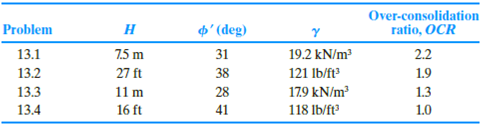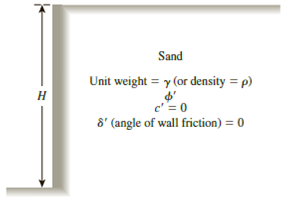Chapter 13, Problem 13.2PPrinciples of Geotechnical Enginee...

9th Edition
Braja M. Das + 1 other
ISBN: 9781305970939

Solutions

Chapter
SectionPrinciples of Geotechnical Enginee...

9th Edition
Braja M. Das + 1 other
ISBN: 9781305970939
Textbook Problem

13.1 through 13.4 Figure 13.39 shows a retaining wall that is restrained from yielding. For each problem, determine the magnitude of the lateral earth force per unit length of the wall. Also, find the location of the resultant, z ¯ , measured from the bottom of the wall.Figure 13.39

To determine

Find the magnitude of the lateral earth forces Po per unit length of the wall and find the location z¯ of the resultant measured from the bottom of the wall.

Explanation

Given information:

The height (H) of the retaining wall is 27 ft.

The soil friction angle ϕ is 38°.

The angle of wall friction δ is 0.

The unit weight γ of the sand is 121lb/ft3

The over-consolidation ratio (OCR) is 1.9.

Calculation:

Calculate the earth pressure coefficient (Ko) using the relation.

Ko=(1sinϕ)(OCR)sinϕ

Substitute 38° for ϕ and 1.9 for OCR.

Ko=(1sin38°)(1.9)sin38°=0.38434×1.4846=0.570

Calculate the total force per unit length (Po) using the relation.

Po=12KoγH2

Substitute 0

Still sussing out bartleby?

Check out a sample textbook solution.

See a sample solution

The Solution to Your Study Problems

Bartleby provides explanations to thousands of textbook problems written by our experts, many with advanced degrees!

Get Started

Explain the differences among data, information, and a database.

Database Systems: Design, Implementation, & Management

When are most liabilities recognized?

Cornerstones of Financial Accounting

What would the divider setting be to scribe a 3/4"-diameter circle?

Precision Machining Technology (MindTap Course List)

What is strategy?

Management Of Information Security

How do volatile and nonvolatile memory differ? (290)

Enhanced Discovering Computers 2017 (Shelly Cashman Series) (MindTap Course List)